Next: Generalized -MDPs Up: -MDPs: Learning in Varying Previous: Q-learning in Generalized MDPs

# MDPs in Varying Environments

In this section we propose an extension of the MDP concept, where transition probabilities are allowed to vary by time. However, without restrictions, such a model would be too general to establish useful theorems. Therefore we restrict ourselves to cases when the variation over time remains small.

We say that the distance of two transition functions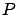and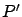is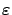-small (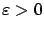), if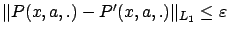for all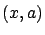, i.e.,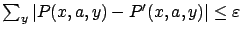for alland subscript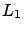denotes thenorm. (Note that for a given stateand action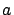,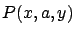is a probability distribution over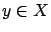.)

A tuple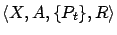is an-stationary MDP [Kalmár et al.(1998)] with, if there exists an MDP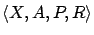(called the base MDP) such that the difference of the transition functionsand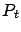is-small for all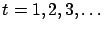.

The simplest example of an-MDP is possibly an ordinary MDP, superimposed by additive noise in its transition function as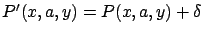in each step, where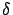is a small amount of noise. A more general case will be discussed within the event-learning framework.

In the ordinary MDP model, the dynamic programming operator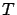is used to find the optimal value function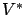.at time step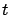is given by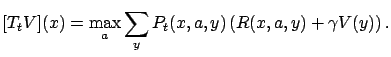(4)

Of course, the iteration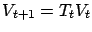does not necessarily have a fixed point. The most that we can expect to find is a close approximation of the optimal value function of the base MDP, i.e., a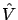such that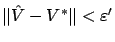with some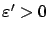.1

In Section 3.2 we show that such an approximation with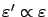can be found (Theorem 3.3).

SubsectionsNext: Generalized -MDPs Up: -MDPs: Learning in Varying Previous: Q-learning in Generalized MDPs